# Slopes of Curves

## Slope – Understanding the Term

In mathematics, slope is a number that describes steepness and direction of a line. In most cases it is denoted by letter m. If slope is positive then the line is increasing, which means it goes up as we move from left to right. If slopei s negative then the line is decreasing, which means it goes down from left to right. If the line is horizontal then it has slope 0. Vertical lines have undefined slope.

To calculate slope we need to find ratio between vertical change and horizontal change, which is often described as ‘rise over run’ (rise is vertical and run is horizontal). Since on most graphs vertical is shown by y-axis and horizontal by x-axis then we define slope as ‘change in y’ divided by ‘change in x’, or as you might find in many books, since change is denoted by ? (delta) then slope m is:

If we are given two points A(x1,y1) and B(x2,y2) then using the definition above and understanding that ‘change’ is actually the difference between the two values we get to the general formula for slope of a line which is:

In this example we have a line passing through points B (0,-1) and C (1,0), so using the formula above the slope is: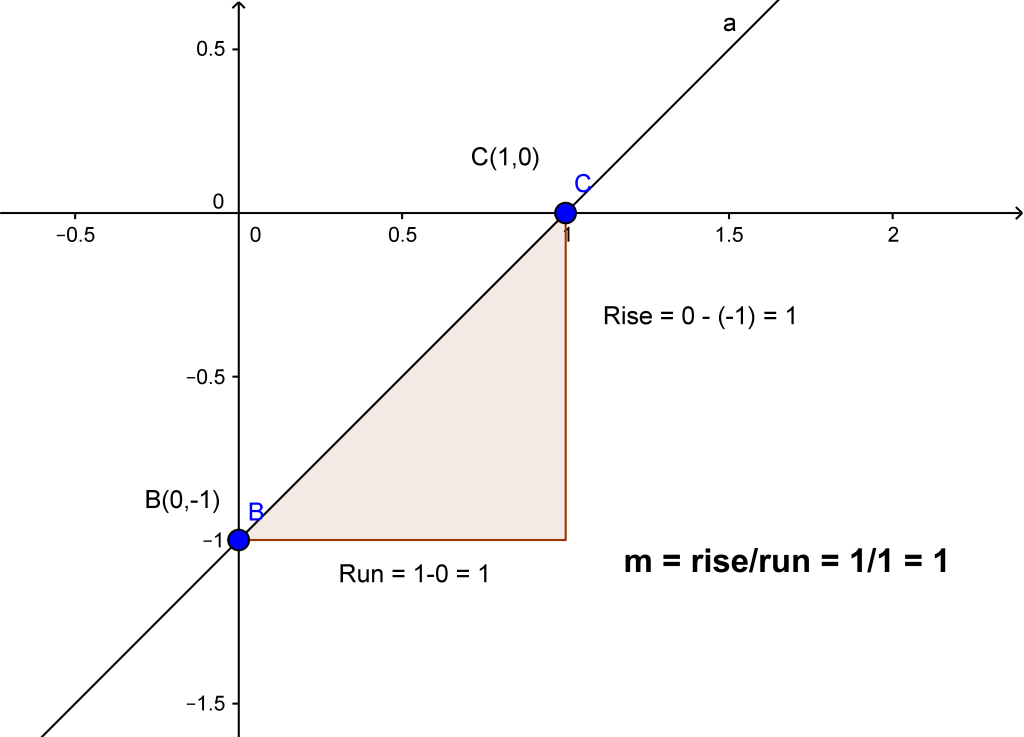Simple. But what about slopes of curves? Stay tuned.

## Introducing Slope of a Curve

We saw earlier that slope describes ratio of changes or rate of change as you might find. Now this is pretty straightforward if we’re talking about constant rate of change which graphed by a straight line. How do we find rate of change when the ratio is different at different points? These types of scenarios are described by curves. That’s why when we talk about slope of a curve we’re actually reffering to slope of a tangent line of that curve at one point. However, in some cases we’re asked to find average rate of change. That is when we need to find slope of a secant line of the curve. This one is easier so let’s examine it first.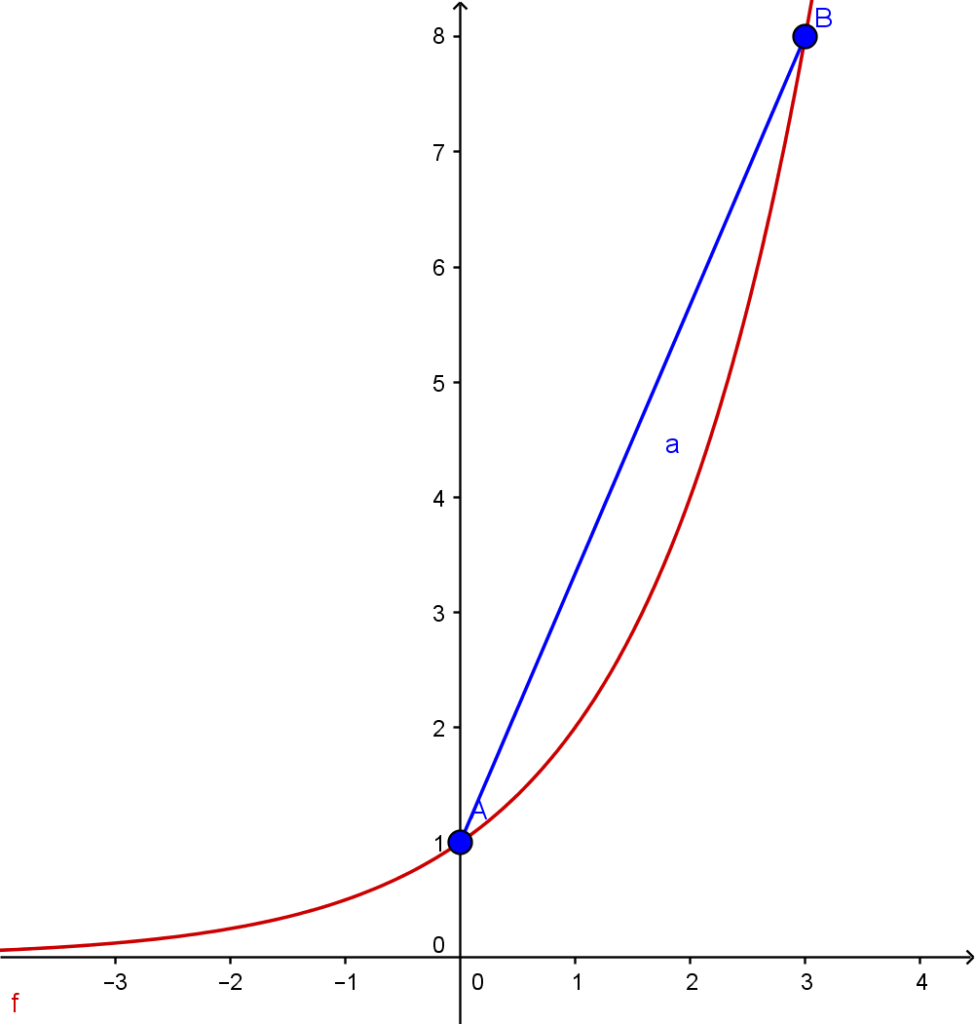Let’s say we need to find average rate of change of this red curve on interval (0,3), which means from x=0 to x=3. This means we need to find slope of a secant AB, where points are A (0,1) and B(2, 8). Here we just apply the slope of a line formula to get:

As simple as it gets. Now let’s talk about the more interesting subject. As I said before, in most cases by slope of a curve we mean slope of a tangent line of that curve. Now you might ask how can this be, since tangent line is a straight line, so it is pretty different than the curve, but let’s take a look.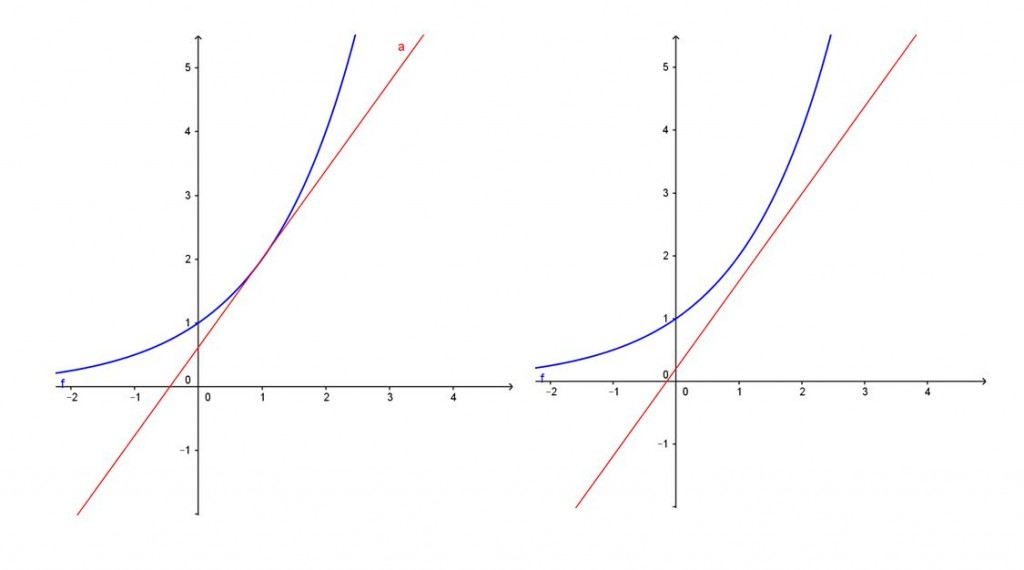On the left side we have a tangent line of the same curve we had above. On the right side we have a line which is parallel to the tangent line which means they have the same slope. Now let’s zoom in on the picture on the right side: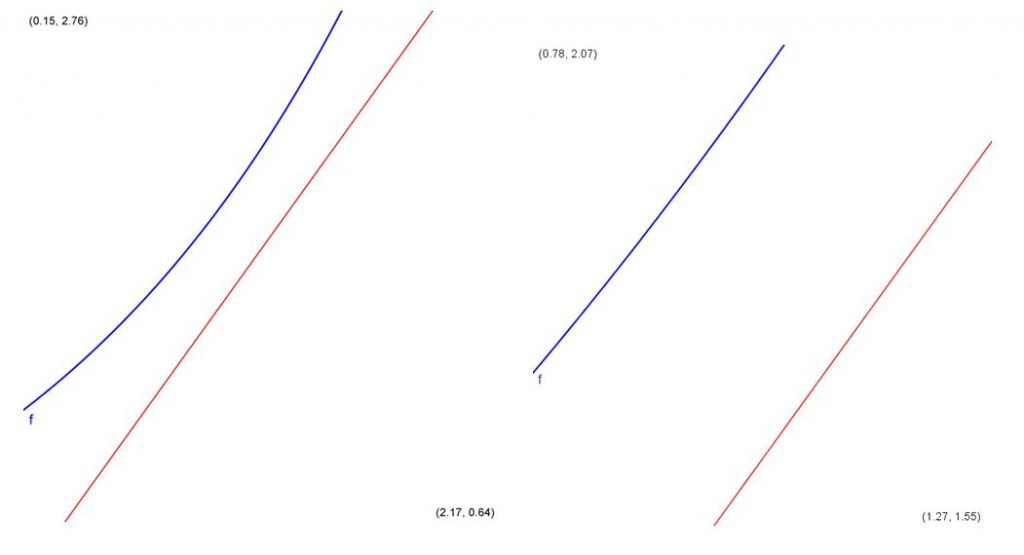As you can see, if we zoom in close enough, the curve actually get’s parallel to the tangent line. This is because a curve is nothing more than a collection of points which are close enough to each other, and as we know, a line is defined by exactly two points. So if we get close enough, we might as well get to those two points where a curve becomes a line, and that line would have exactly the same slope as the tangent line. But enough about theory, let’s take a look at how we can actually find slope of a curve (or slope of a tangent of a curve).

## Intuitive way to find slope of a tangent to a curve

Let’s get straight to the example. Let f(x) = x² – 2x + 1. Say we need to find tangent of this curve at point A (2, 1). The first thing we might try is numerical approach. Now I know that this slope is supposed to be m = 2 (we’ll talk about finding exact slope in a minute) but let’s see if we can get close to that. First we’ll find slope of a secant line AB where B will be at (1,0). Using the formula for slope we easily calculate: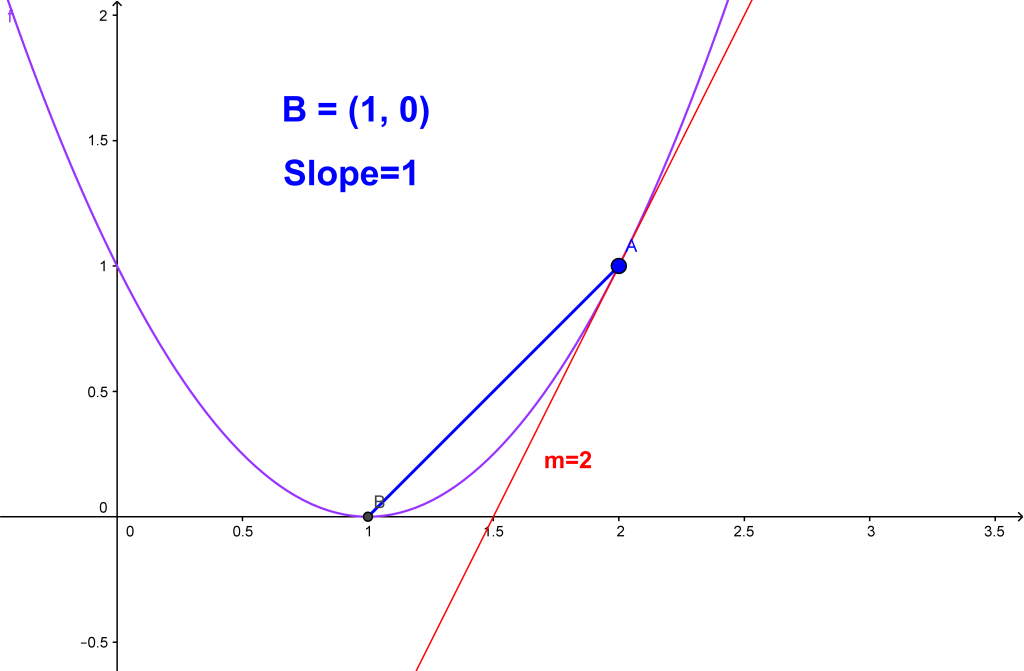Now let’s move point B a little closer to point A and calculate again: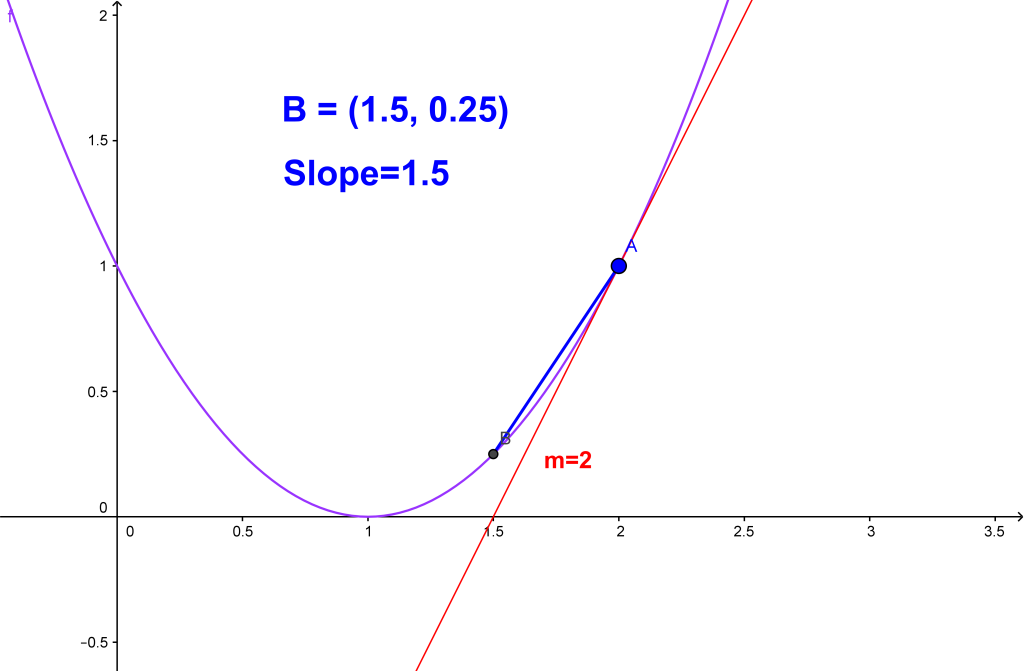As you can see, we moved point B closer to point A and thus we got our slope to be 1.5, which is closer to 2 (the exact slope of the tangent line).

Now observe this animation and see what happens whith the slope of the secant as we move point B closer and closer to point A: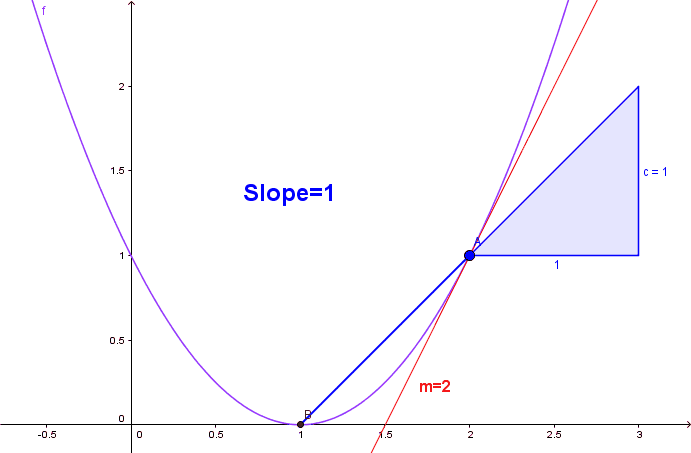Wow! As you can see, if we get the difference between points A and B to be small enough, we get the slope to be as close as possible to the exact slope m=2. But can we get it to be exact? Not without the help from the limits.

## First Principles a.k.a. ‘The Delta Method’

So what we found out is that, to get the exact slope, we need the difference between two points of a secant line to be zero. But if we do that, we will get 0/0 indeterminate. So what we use instead is limits. Let h be the difference in x-values of two points A and B (we’re using the same example as above). Then y-values are f(x) and f(x+h) since h is the difference. Since slope is change in y over change in x this gives us that:

And since we need the difference to be zero, we let h0 so finally we get:

This is called differentiation from first principles and the limit above is the limit definition of derivative. To give you an illustration of it let’s take a look at the graph of a function sin(x). The derivative of that function is cos(x) since .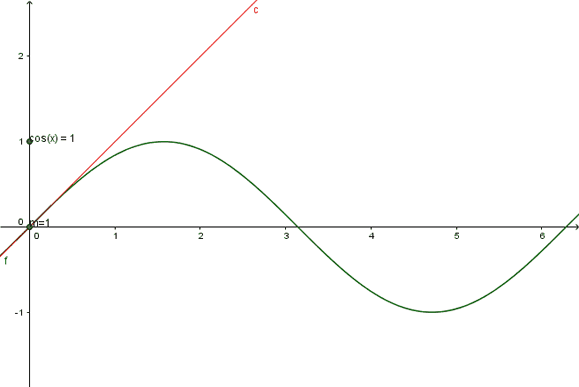The green curve is sin(x) and you can see that the slope of that curve m at any point x is the same value as cos(x). That’s why it leaves the trace of a cosine function cos(x). This tells us that, in order to find slope of a curve at some point, we need to find derivative of that curve.

Example: Find slope of a curve  at point A (1,2).

Solution: First, using limit definition of a derivative, let’s find derivative of this function:

After canceling the fraction by h we get:

And substitute 0 instead of h now to get:

We’re looking for slope at point (0,-1) , so put 0 instead of x to find slope:

Finding Slopes of Curves Using Derivatives

Using first principles approach is accurate but slow. Since we use derivatives to find slopes then let’s take a look at some basic rules of differentiation to speed things up. Here’s the table of derivatives to use:

There are some others but these will be just enough for now. There are also rules of differentiation:

Multiplication by constant:

Sum rule:

Product rule:

Once you get used to these rules then finding derivatives, and by that also finding slopes becomes fast, fun and easy.

Example: Find the slope of a curve f(x) = x² + x³ at point A (1,2).

Solution: First find derivative of a function f(x) using the rules above and table of derivatives.

Using the sum rule we have that:

And using the derivative we get that:

Since we need to find slope at point A (1,2) then put 1 instead of x to get:

[box type=”success” align=”” class=”” width=””]For more information and examples check out the lesson on Overview & Slopes of Curves and More On Slopes of Curves in our AP Calculus AB course.[/box]

SPECIAL OFFER!

SUCCESS8